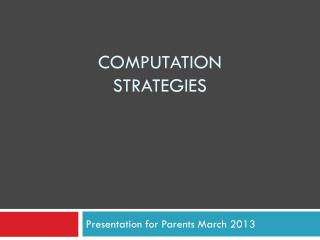DownloadDownload PresentationComputation STRATEGIES

# Computation STRATEGIES

Download Presentation## Computation STRATEGIES

- - - - - - - - - - - - - - - - - - - - - - - - - - - E N D - - - - - - - - - - - - - - - - - - - - - - - - - - -
##### Presentation Transcript

1. ComputationSTRATEGIES Presentation for Parents March 2013

2. Flexible Thinking • There are many ways to solve problems. • The more ways we solve a problem, the deeper our understanding of the mathematics. • When we ask "How would you do that?" or "Can you show me another way?" we are helping children understand math better. • Encourage children to use what they know to solve problems. • Traditional algorithms involving lining up columns rely heavily on memorizing procedures and can work against developing number sense. • 85% of all calculations we do involve mental math.

3. Addition and Subtraction FactsGrades 1, 2 and 3 Students will learn mental mathematics strategies to determine basic addition and related subtraction facts to 18.

4. Doubles 1+1=2 2+2=4 3+3=6 4+4=8 5+5=10 6+6=12 7+7=14 8+8=16 9+9=18

5. DOUBLES +1DOUBLES -1 FOR 5+6= THINK 5+5 = 10 SO…. 5+6 = 11 FOR 4+5= THINK 5+5 = 10 SO…. 4+5 = 9

6. Commutative Property(Flip) FOR 3+9= THINK 9+3 = 12 SO…. 3+9 = 12

7. Friendly Number 10 1+99+1 2+88+2 3+77+3 4+6 6+4  5+5

8. Make 10 When 1 of the numbersyou are addingis 7,8 or 9 Go to 10 And add the rest For 8+5 Think 8 + 2 + 3 10 + 3 = 13 So… 8+5 = 13

9. Counting on For 6+2 = Start at the largestnumber and count forwards: 6…7,8 So 6+2 = 8 ______________________ 6 7 8

10. Counting Back For 6-2 = Start at the largestnumber and count backwards: 6…5,4 So 6-2 = 4 ___________ 4 5 6

11. Think Addition for Subtraction For 12- 5= Think 5 + __ = 12 So 12 – 5 = 7 ______________________ 5 10 12

12. Zero Property Anynumber + 0 or -0 Remains the same 5+0=5 9-0=9

13. Number Families If I know that 3+4=7 I also know that 4+3=7 7-4=3 7-3=4

14. 0,1,2/Doubles/ Near Doubles/Make 10

15. Computation Strategies for Addition and Subtraction of Large Numbers • Students will use personal strategies to add and subtract numbers in problem solving situations.

16. Place Value Decomposing Numbers 334 + 419 = (300 + 400) + (30 + 10) + (4 + 9) 700 + 40 + 13 753

17. Place Value Place Value Left to Right 334 + 419 Add the 100s 700 Add the 10s 40 Add the 1s + 13 753

18. Blank Number Line Number Line  334 + 419 = +300 +30 +1 +3 419 719 749 750 753

19. Think Addition for Subtraction 828 - 729 = 70+28+1=99 +1 +70 +28 729 730 800 828

20. Multiplication and Divison FactsGrades 3-6 Students will demonstrate and apply different mental mathematical strategies to develop recall of basic multiplication facts to 9 x 9 and related division facts.

21. Doubling and Halving Doubling and Halving *halve one number and double the othernumber 15 x 4 = 30 x 2= So 30 x 2 = 60

22. Skip Counting from a Known Number Skip Counting from a Known Number 6 X 7 = I know that 5 X 7 = 35 So…6 X 7 = 35 + 7 = 42

23. Distributive Property 6 X 7 (6 X 5 =)+(6 X 2 =) 30 + 12 42

24. Commutative Property 6 X 7= 7x6=

25. Arrays 3x2= 2x3= 6÷3=2 6÷2=3

26. Fact Families 6 X 7=42 7x6=42 42÷6=7 42÷7=6

27. Think Multiplication for Division 20÷5= Think how many groups of 5 are in 20? Or 5x?=20

28. Computation Strategies for Multiplication • Students will demonstrate an understanding of multiplication by using different strategies to determine answers.

29. Repeated Addition 15 x 6 = Add15 six times 15 + 15 + 15 + 15 + 15 + 15 = 30 + 30 + 30 90

30. Place Value(Distributive Property) 15 x 6 (10 x 6) + (5 x 6) 60 + 30 = 90

31. Place Value(Left to Right) Place Value Left to Right 15 x 6 60 +30 90

32. Computation Strategies for Division • Students will demonstrate an understanding of division by using different strategies to determine answers.

33. Division Place Value 4 136 30 -120 + 4 16 34 -16 0

34. For More information Pembina Trails School Division http://www.pembinatrails.ca/program/earlyyears/Link%205/Numeracy.html Manitoba Education and Literacy http://www.edu.gov.mb.ca/k12/parents/index.html

35. A Star and A Wish I appreciated learning……. I wish I could lean more about…..